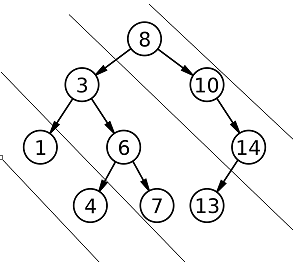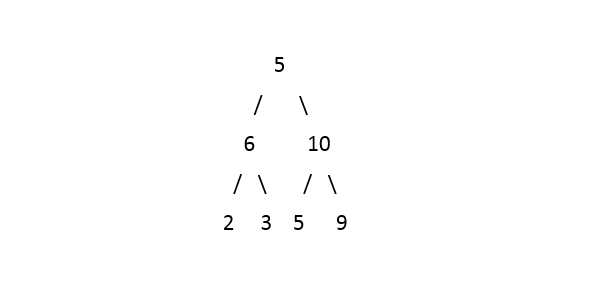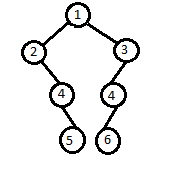14

# Diagonal Sum

Difficulty: EASYContributed By
Ayush Thakur
Avg. time to solve
23 min
Success Rate
75%

Problem Statement
Suggest Edit

#### Diagonals of the binary tree are :#### Diagonal 3 : 1 4

``````Example :

For the given binary tree :
````````````Output : 24 14 2

Explanation: Rightmost diagonal contains elements {5, 10 , 9} its sum is 24, middle diagonal contains elements {6, 3, 5} its sum is 14, leftmost diagonal contains elements {2}. Thus the answer should be “24 14 2”.
``````
##### Input Format :
``````The only line of the test case contains elements in the level order form. The line consists of values of nodes separated by a single space. In case a node is null, we take -1 on its place.

For example, the input for the tree depicted in the below image would be :
````````````1
2 3
4 -1 5 6
-1 7 -1 -1 -1 -1
-1 -1
``````

#### Explanation :

``````Level 1 :
The root node of the tree is 1

Level 2 :
Left child of 1 = 2
Right child of 1 = 3

Level 3 :
Left child of 2 = 4
Right child of 2 = null (-1)
Left child of 3 = 5
Right child of 3 = 6

Level 4 :
Left child of 4 = null (-1)
Right child of 4 = 7
Left child of 5 = null (-1)
Right child of 5 = null (-1)
Left child of 6 = null (-1)
Right child of 6 = null (-1)

Level 5 :
Left child of 7 = null (-1)
Right child of 7 = null (-1)

The first not-null node (of the previous level) is treated as the parent of the first two nodes of the current level. The second not-null node (of the previous level) is treated as the parent node for the next two nodes of the current level and so on.
The input ends when all nodes at the last level are null (-1).
``````
##### Note :
``````The above format was just to provide clarity on how the input is formed for a given tree.
The sequence will be put together in a single line separated by a single space. Hence, for the above-depicted tree, the input will be given as:

1 2 3 4 -1 5 6 -1 7 -1 -1 -1 -1 -1 -1
``````
##### Output Format :
``````Print the only line of output will contain a list containing diagonal sums from right diagonal to left diagonal.
``````
##### Note :
``````You are not required to print the output explicitly, it has already been taken care of. Just implement the function.
``````
##### Constraints :
``````0 <= N <= 10^5
0 <= node.data <= 10^9

Time Limit : 1 sec
``````
##### Sample Input 1 :
``````1 2 3 -1 4 4 -1 -1 5 6 -1 -1 -1 -1 -1
``````
##### Sample Output 1:
``````4 15 6
``````
##### Explanation For Sample Input 1 :
``````The input binary tree will be represented as
````````````From the above representation, the rightmost diagonal contains elements {1, 3}, the middle diagonal contains elements {2, 4, 4, 5} and leftmost diagonal elements are {6}. Thus the list should contain “4 15 6”.
``````
##### Sample Input 2:
``````1 2 3 -1 -1 -1 -1
``````
##### Sample Output 2 :
``````4 2
``````Console